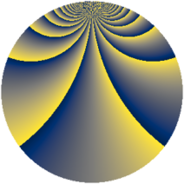# Properties

 Label 2850.2.csLevel $2850$ Weight $2$ Character orbit 2850.cs Rep. character $\chi_{2850}(13,\cdot)$ Character field $\Q(\zeta_{180})$ Dimension $4800$ Sturm bound $1200$

# Learn more about

## Defining parameters

 Level: $$N$$ $$=$$ $$2850 = 2 \cdot 3 \cdot 5^{2} \cdot 19$$ Weight: $$k$$ $$=$$ $$2$$ Character orbit: $$[\chi]$$ $$=$$ 2850.cs (of order $$180$$ and degree $$48$$) Character conductor: $$\operatorname{cond}(\chi)$$ $$=$$ $$475$$ Character field: $$\Q(\zeta_{180})$$ Sturm bound: $$1200$$

## Dimensions

The following table gives the dimensions of various subspaces of $$M_{2}(2850, [\chi])$$.

Total New Old
Modular forms 29184 4800 24384
Cusp forms 28416 4800 23616
Eisenstein series 768 0 768

## Trace form

 $$4800q - 24q^{7} + O(q^{10})$$ $$4800q - 24q^{7} - 96q^{22} - 192q^{23} - 24q^{25} + 240q^{29} + 96q^{33} + 360q^{43} + 192q^{47} + 72q^{53} - 72q^{55} - 24q^{57} + 240q^{59} + 24q^{60} - 48q^{62} - 144q^{67} - 24q^{68} - 48q^{70} - 72q^{73} + 48q^{78} + 96q^{82} + 168q^{83} + 384q^{85} + 96q^{87} + 96q^{92} + 48q^{95} + 192q^{97} + 192q^{98} + O(q^{100})$$

## Decomposition of $$S_{2}^{\mathrm{new}}(2850, [\chi])$$ into newform subspaces

The newforms in this space have not yet been added to the LMFDB.

## Decomposition of $$S_{2}^{\mathrm{old}}(2850, [\chi])$$ into lower level spaces

$$S_{2}^{\mathrm{old}}(2850, [\chi]) \cong$$ $$S_{2}^{\mathrm{new}}(475, [\chi])$$$$^{\oplus 4}$$$$\oplus$$$$S_{2}^{\mathrm{new}}(950, [\chi])$$$$^{\oplus 2}$$$$\oplus$$$$S_{2}^{\mathrm{new}}(1425, [\chi])$$$$^{\oplus 2}$$